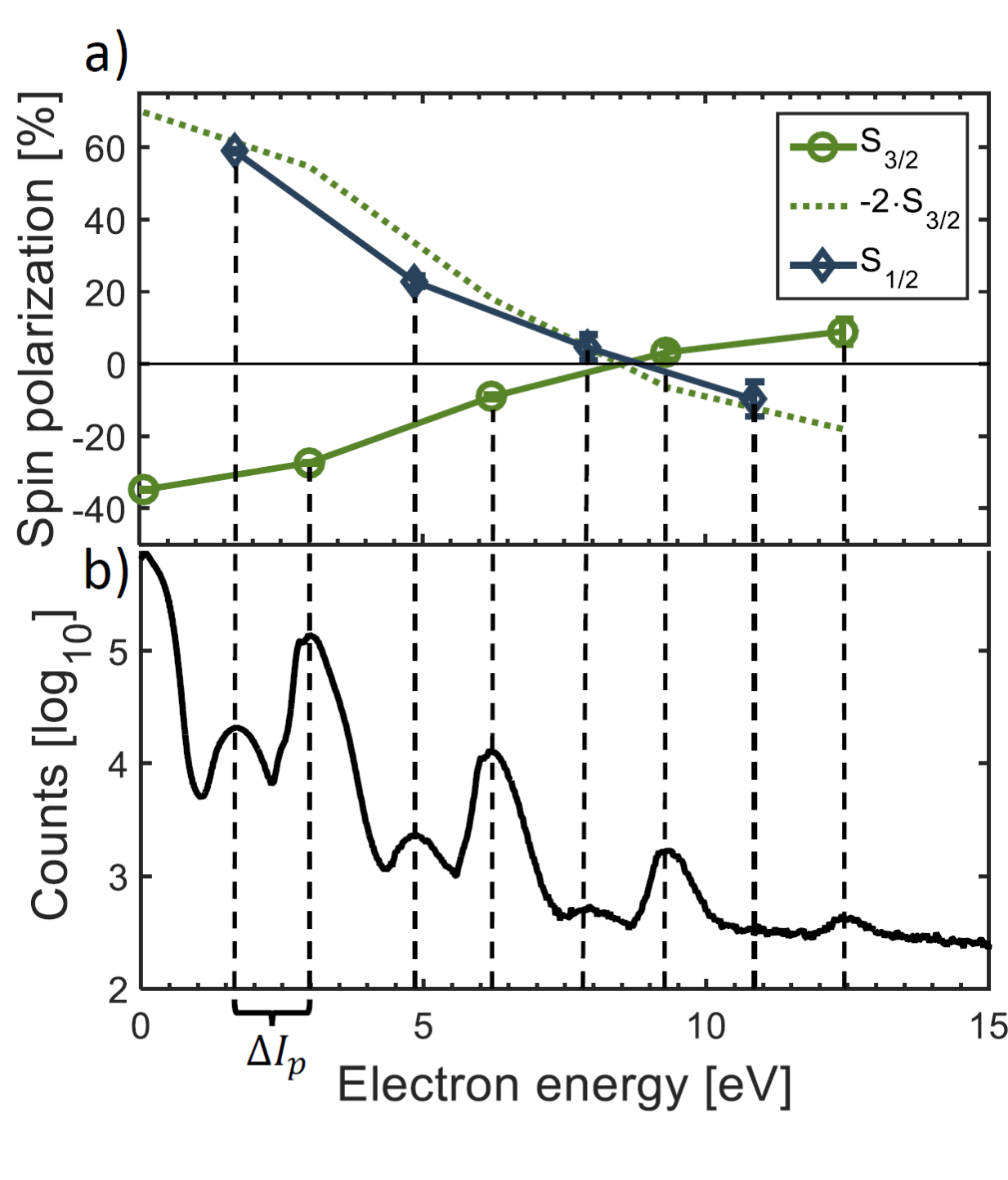﻿ Atomic Physics - Laser

Spin and Angular Momentum in Strong-Field Ionization

As demonstrated previously by Hartung et al., irradiating Xenon with circularly polarized laser pulses creates an energy-dependent average spin orientation i.e. spin polarization of the released electrons. The ionization occurs mainly from two angular momentum states, 5p j=3/2 and 5p j=1/2, which are separated by 1.3 eV in binding energy.

Here, we report on the first angular-momentum resolved measurement of the spin polarization in strong-field ionization. Xenon was irradiated by circularly polarized light at a wavelength of 395 nm, the electrons were guided to a Mott polarimeter. At this photon energy of 3.14 eV, the above-threshold ionization (ATI) peaks connected to the j=3/2 and j=1/2 states are clearly separated in the electron energy distribution (see Figure b)). Confirming theoretical predictions, these two combs of ATI peaks show opposite spin polarizations. The magnitude of the spin polarization is a factor of two higher for the j=1/2 than for the j=3/2 state. The distinction between both angular momentum states allows for the creation of highly polarized electron bursts as one of many possible applications.Energy-dependent spin polarization S for ionization of xenon by circularly polarized laser pulses a) Measured spin polarization for the 5p j = 3/2 (green circles) and 5p j = 1/2 (blue symbols) states of Xe. The full lines are drawn to guide the eye. The green dotted line is obtained by flipping the green line at the horizontal axis and stretching it by a factor of two vertically. This is what one would expect from theory. b) Electron energy distribution measured on one of the Mott-polarimeter’s detectors. The comb of high peaks corresponds to the j = 3/2 state, the comb of lower peaks is shifted by 1.3 eV and corresponds to the j = 1/2 state of Xe.

Publication:

Spin and Angular Momentum in Strong-Field Ionization
D. Trabert, A. Hartung, S. Eckart, F. Trinter, A. Kalinin, M. Schöffler, L. Ph. H. Schmidt, T. Jahnke, M. Kunitski, and R. Dörner

DOI: 10.1103/PhysRevLett.120.043202

See online:

Locally hosted pdf from publication